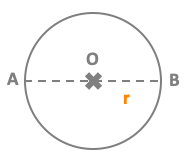# Area of a circleArea A = π x r²

with π (number pi) approximately equal to 3.14
Radius = AO = OB = r
Diameter = AB = 2 r

## Example

Take a circle with radius r = 5 cm
Area A of the circle = π x r² ≈ 3.14 x 5² ≈ 78.5 cm²

## Definition of a circle

A circle is a closed shape formed by tracing a point that moves in a plane such that its distance from a given point is constant.
When a set of all points that are at a fixed distance from a fixed point are joined then the geometrical figure obtained is called circle.
Radius is the fixed distance between the center and the set of points. It is noted "r".
Diameter is a line segment, having boundary points of circles as the endpoints and passing through the center.

A circle is a round shaped figure that has no corners or edges.
A circle is a shape consisting of all points in a plane that are at a given distance from a given point : the centre.
A circle is the set of all points in a plane that are equidistant from a given point called the center of the circle.

The circumference of a circle is the length around the circle.
The circumference of the circle is equal to the length of its boundary.

The area of a circle is the amount of space enclosed within the boundary of a circle.
The area of a circle is pi times the radius squared: Area A = π x r².

### A chord

A chord is an line segment that has any two points on the circumference as its end-points. A chord always lies inside a circle.
The chord of a circle can be defined as the line segment joining any two points on the circumference of the circle.
A chord of a circle refers to a straight line segment whose endpoints both lie on the circle.

### A diameter

A diameter of a circle is a chord that contains the center of the circle.
A line that passes through the center and its endpoints lie on the circle is called the diameter of a circle.
The diameter of a circle is a line segment that passes through the center of a circle and has two endpoints at the circumference.

### A secant

A secant is a line that intersects the circle at two points.
A secant is line that cuts a circle at two points.
A secant is a line that intersects with a circle at two different points.

### A tangent

A tangent to a circle is a line that intersects the circle at only one point. The point where the tangent intersects the circle is called the point of tangency.
A tangent is a line that intersects a circle at exactly one point.

## Differences between a circle and a disc

A circle is the boundary of a disc.
A disc (also spelled disk) is the region in a plane bounded by a circle.
A disc with center O and radius r is the surface bounded by the circle with center O and radius r.